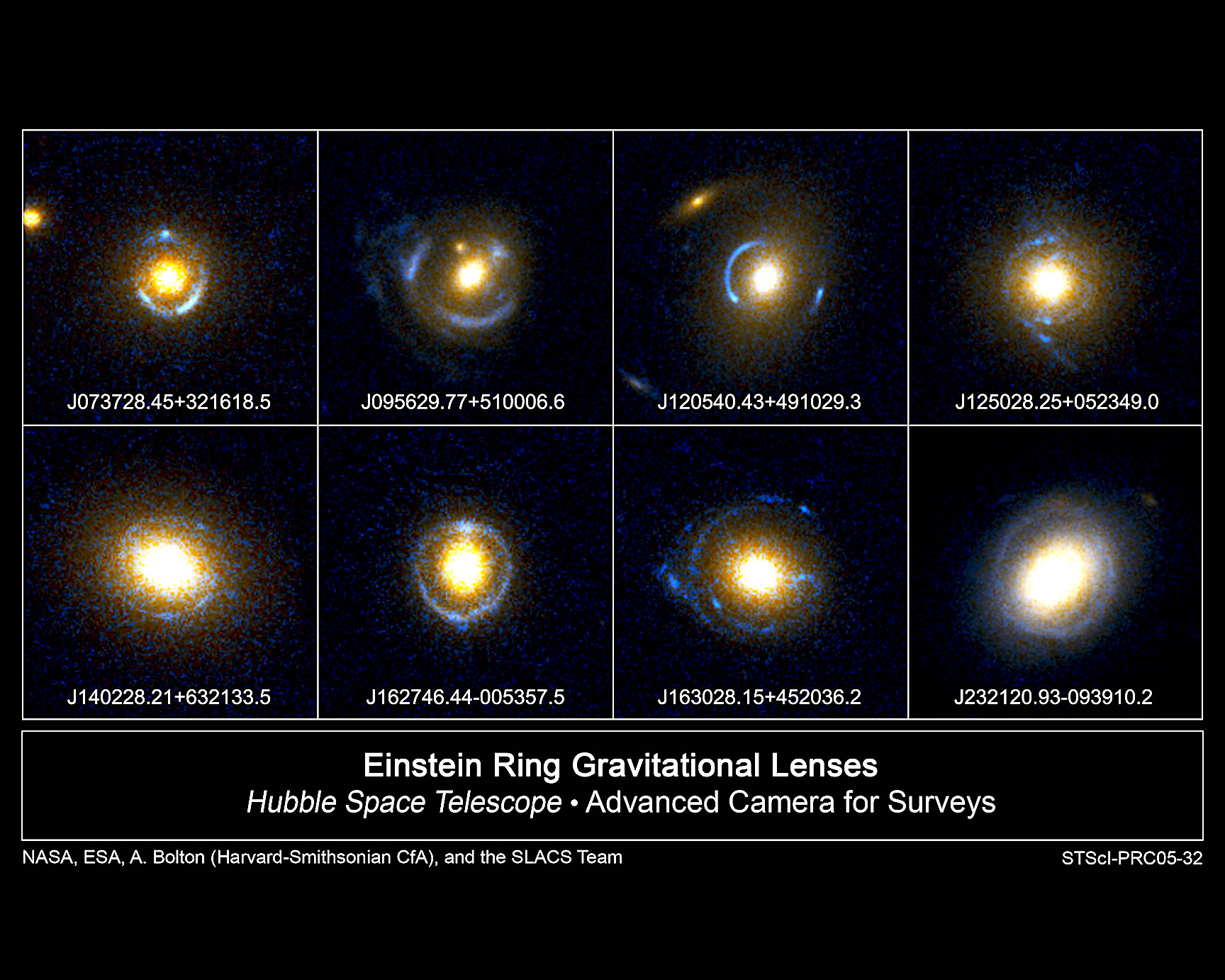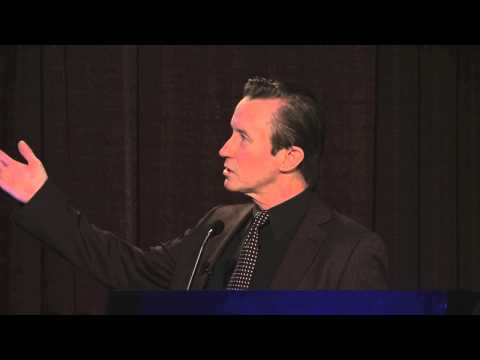# * God particles (without Nobel Prize) and Vacuum.

socratus
#1
God- particle (without Nobel Prize) and Vacuum.
==..
To discover so-called God - particle ( Nobel Prize in 2013)
was needed two conditions : deep vacuum* and* high energy.
But the CERN* project says:
if energy is higher and vacuum is deeper then it is possible
to discover some kind of a* new* particles.
Question: what is the deepest vacuum in the Universe?
the deepest vacuum in the Universe is cosmic zero vacuum T=0K.
Question: what can be the highest energy?
the cosmic zero vacuum T=0K continuum is itself some kind
of infinite energy continuum.
#
What is zero vacuum T=0K ?
a)
Zero vacuum has the same T=0K temperature as ''ideal gas''
and therefore i use ''Theory of ideal gas'' to understand
zero vacuum continuum.
According to J.Charle's law ( 1787) : when the temperature falls 1 degree,
the volume decreases 1/273. And when the temperature reaches -273
degree the volume disappears and particles become " flat figures ".
Charle's law* was confirmed by other physicists: Gay-Lussac, Planck,
Nernst, Einstein .
These " flat figures " have the geometrical form of a circle, as from
all flat figures the circle has the most optimal form.
So, the primary elementary quantum particle has geometrical form
of membrane-circle: c/d=pi= 3,1415 . . . .
b)
Every vacuum region (R) has the same numbers of
Then every single quantum particle has Boltzmann constant
potential molar-mass* R/ N(a)=k. (virtual particle)
c)
Zero vacuum is some kind of infinite energy (E) with infinite
mass (M) of Boltzmann k-particles. Then every k-particle
has potential energy:* +/- E/M=c^2.* (virtual particle)
In vacuum quantum potential energy has potential impulse h=0.
d)
Heat is result of some kind of chaotic movements of particles.
In thermodynamics the heat is explained by the formula: E=kT (logW)
It means: chaotic movements of molar-masses k- particles create heat.
e)
Energy is result of Plank's (h) "quantum of action" : E=h*f.
In my opinion it means - the potential energy:* +/- E/M=c^2 can
by ''transformation -fluctuation . . .''* jump trough so called
''quantum tunneling phenomena'' and be real particle.
Such vacuum effects were observed.
1) “ Its effects can be observed in various phenomena
(such as spontaneous emission, the Casimir effect, the
van der Waals bonds, or the Lamb shift), and it is thought
to have consequences for the behavior of the Universe
on cosmological scales. “
/ http://en.wikipedia.org/wiki/Vacuum_energy /
2) Chalmers scientists create light from vacuum
http://www.chalmers.se/en/news/Pages/Ch ... acuum.aspx
And Newton wrote:
‘'For the basic problem of philosophy seems to be to discover
the forces of nature from the phenomena of motions
and then to demonstrate the other phenomena from these forces.’'
f)
Without heat the Universe is an Absolute Zero Cold Kingdom.
Without "quantum of action" the Universe is dead continuum.
The interaction between energy and heat created the material
Universe but . . . but until today nobody explained the interaction
between E= h*f and E=kT (logW).
My conclusion.
Vacuum is not nothingness.
If vacuum can somehow create light, particles and heat then it is
possible that universe and existence started from the infinite vacuum
continuum T=0K and not " the famous Higgs boson"* but the
well-known Boltzmann / Planck / Einstein particle is real "God particle"
================
Attached Images
god particle.jpg (6.4 KB, 0 views )

darkbeaver
#2
Quote: Originally Posted by socratusGod- particle (without Nobel Prize) and Vacuum.
==..
To discover so-called God - particle ( Nobel Prize in 2013)
was needed two conditions : deep vacuum* and* high energy.
But the CERN* project says:
if energy is higher and vacuum is deeper then it is possible
to discover some kind of a* new* particles.
Question: what is the deepest vacuum in the Universe?
the deepest vacuum in the Universe is cosmic zero vacuum T=0K.
Question: what can be the highest energy?
the cosmic zero vacuum T=0K continuum is itself some kind
of infinite energy continuum.
#
What is zero vacuum T=0K ?
a)
Zero vacuum has the same T=0K temperature as ''ideal gas''
and therefore i use ''Theory of ideal gas'' to understand
zero vacuum continuum.
According to J.Charle's law ( 1787) : when the temperature falls 1 degree,
the volume decreases 1/273. And when the temperature reaches -273
degree the volume disappears and particles become " flat figures ".
Charle's law* was confirmed by other physicists: Gay-Lussac, Planck,
Nernst, Einstein .
These " flat figures " have the geometrical form of a circle, as from
all flat figures the circle has the most optimal form.
So, the primary elementary quantum particle has geometrical form
of membrane-circle: c/d=pi= 3,1415 . . . .
b)
Every vacuum region (R) has the same numbers of
Then every single quantum particle has Boltzmann constant
potential molar-mass* R/ N(a)=k. (virtual particle)
c)
Zero vacuum is some kind of infinite energy (E) with infinite
mass (M) of Boltzmann k-particles. Then every k-particle
has potential energy:* +/- E/M=c^2.* (virtual particle)
In vacuum quantum potential energy has potential impulse h=0.
d)
Heat is result of some kind of chaotic movements of particles.
In thermodynamics the heat is explained by the formula: E=kT (logW)
It means: chaotic movements of molar-masses k- particles create heat.
e)
Energy is result of Plank's (h) "quantum of action" : E=h*f.
In my opinion it means - the potential energy:* +/- E/M=c^2 can
by ''transformation -fluctuation . . .''* jump trough so called
''quantum tunneling phenomena'' and be real particle.
Such vacuum effects were observed.
1)  Its effects can be observed in various phenomena
(such as spontaneous emission, the Casimir effect, the
van der Waals bonds, or the Lamb shift), and it is thought
to have consequences for the behavior of the Universe
on cosmological scales. 
/ http://en.wikipedia.org/wiki/Vacuum_energy /
2) Chalmers scientists create light from vacuum
http://www.chalmers.se/en/news/Pages/Ch ... acuum.aspx
And Newton wrote:
'For the basic problem of philosophy seems to be to discover
the forces of nature from the phenomena of motions
and then to demonstrate the other phenomena from these forces.'
f)
Without heat the Universe is an Absolute Zero Cold Kingdom.
Without "quantum of action" the Universe is dead continuum.
The interaction between energy and heat created the material
Universe but . . . but until today nobody explained the interaction
between E= h*f and E=kT (logW).
My conclusion.
Vacuum is not nothingness.
If vacuum can somehow create light, particles and heat then it is
possible that universe and existence started from the infinite vacuum
continuum T=0K and not " the famous Higgs boson"* but the
well-known Boltzmann / Planck / Einstein particle is real "God particle"
================

I don,t agree with much that you say Socratus but you do have an interest in physics, so you can,t be a bad person. The universe had no beginning and has no end this was known for long ages past.

Plasma GunsFeb 21, 2017 Space and time do not bend. Gravitational lensing theory is used to explain the arcs of glowing material surrounding some galaxies. They also use it in conjunction with other theories to help deal with puzzling observations. One of those puzzles involves the so-called expanding Universe. Combining dataStephen J. Crothers on Non-existence of Black Holes & The Failure of General Relativity.

Danbones
#3
The idea that things get bigger the faster you go, like the gas bill for my boat...gee, who the hell thought that up?

socratus
#4
''darkbeaver''
yeah,
''The universe has no beginning and has no end
this was known for long ages past.'' . . . . but . . .
but what is physical parameters of this ''no beginning - no end'' ?

========

Danbones
#5
But of course, then you have GAWD, and he because he is a total TROLL, he says "Screw physics!!!, We're doin' KOOKOO!!!and so we get GENOCIDAL B*TF*CK KooKoo.

I guess we need the genocidal KooKoo on the destruct cycle now so we get to create a brave new world in the afterlaugh.
Last edited by Danbones; Apr 25th, 2018 at 05:37 AM..

socratus
#6
Today the concept of* an* ''Ideal Gas'' is adopted
in scientific community as an abstract model in
thermodynamics but if ''ideal gas'' and zero vacuum have
equal temperature then situation is different.
#
In 1854, . . . Helmholtz realized that the laws of
thermodynamics could be applied to the universe as a whole,
meaning that everything around us, including the stars and
galaxies, would eventually have to run down.
/ Book: Parallel Worlds , page 289, by Michio Kaku /
=============